## space-time dual classical circuits

Austen Lamacraft (Cambridge) and Pieter Claeys (Dresden)

austen.uk/#talks for slides

## Dual unitaries and their phenomenology

### Expectation values

• Evaluate $\bra{\Psi}\mathcal{O}\ket{\Psi}=\bra{\Psi_0}\mathcal{U}^\dagger\mathcal{O}\mathcal{U}\ket{\Psi_0}$ for local $\mathcal{O}$

### Folded picture

• After folding, lines correspond to two indices / 4 dimensions

### Unitarity in folded picture

• Circle denotes $\delta_{ab}$

### $\bra{\Psi}\mathcal{O}\ket{\Psi}$ in folded picture

• Emergence of “light cone”

### Reduced density matrix

• Expectation values in region $A$ evaluated using reduced density matrix

$$\rho_A = \operatorname{tr}_{\bar A}\left[\ket{\Psi}\bra{\Psi}\right]=\operatorname{tr}_{\bar A}\left[\mathcal{U}\ket{\Psi_0}\bra{\Psi_0}\mathcal{U}^\dagger\right]$$

### Toy model

• For a Bell pair consisting of qubits at sites $m$ and $n$:

• If $n\in A$, $m\in\bar A$, $\rho_A$ has factor $\mathbb{1}_n$.

• If $m, n\in A$ they contribute a factor $\ket{\Phi^+}_{nm}\bra{\Phi^+}_{nm}$ (pure)

• Only first case contributes to $S_A = \min(4\lfloor t/2\rfloor, |A|)$ bits

### $\rho_A$ via dual unitarity

• 8 sites; 4 layers

• $\rho_A$ is unitary transformation of

$$\mathbb{1}\otimes\mathbb{1}\otimes\mathbb{1}\otimes\mathbb{1}\otimes\mathbb{1}\otimes\mathbb{1}\otimes\mathbb{1}\otimes\mathbb{1}$$

### Shallower…

• $\rho_A$ is unitary transformation of

$$\mathbb{1}\otimes\mathbb{1}\ket{\Phi^+}\bra{\Phi^+}\otimes\ket{\Phi^+}\bra{\Phi^+}\otimes\mathbb{1}\otimes\mathbb{1}$$

### General case

• RDM is unitary transformation of

$$\rho_0=\overbrace{\frac{\mathbb{1}}{2}\otimes \frac{\mathbb{1}}{2} \cdots }^{t-1} \otimes\overbrace{\ket{\Phi^+}\bra{\Phi^+} \cdots }^{N_A/2-t+1 } \otimes \overbrace{\frac{\mathbb{1}}{2}\otimes \frac{\mathbb{1}}{2} \cdots }^{t-1}$$

• RDM has $2^{\min(2t-2,N_A)}$ non-zero eigenvalues all equal to $\left(\frac{1}{2}\right)^{\min(2t-2,N_A)}$

• Converse – maximal entanglement growth implies dual unitary gates – recently proved by Zhou and Harrow (2022)

### Thermalization

• After $N_A/2 + 1$ steps, reduced density matrix is $\propto \mathbb{1}$

• All expectations (with $A$) take on infinite temperature value

### The dual unitary family

• $4\times 4$ unitaries are 16-dimensional

• Family of dual unitaries is 14-dimensional

• Includes kicked Ising model at particular values of couplings

• Dual unitaries not “integrable” but have enough structure to allow many calculations

### Floquet theory: kicked Ising model

• Time dependent Hamiltonian with kicks at $t=0,1,2,\ldots$.

\begin{aligned} H_{\text{KIM}}(t) = H_\text{I}[\mathbf{h}] + \sum_{m}\delta(t-n)H_\text{K}\\ H_\text{I}[\mathbf{h}]=\sum_{j=1}^L\left[J Z_j Z_{j+1} + h_j Z_j\right],\qquad H_\text{K} &= b\sum_{j=1}^L X_j, \end{aligned}

• “Stroboscopic” form of $U(t)=\mathcal{T}\exp\left[-i\int^t H_{\text{KIM}}(t’) dt’\right]$

\begin{aligned} U(n_+) &= \left[U(1_+)\right]^n,\qquad U(1_-) = K I_\mathbf{h}\\ I_\mathbf{h} &= e^{-iH_\text{I}[\mathbf{h}]}, \qquad K = e^{-iH_\text{K}} \end{aligned}

### KIM as a circuit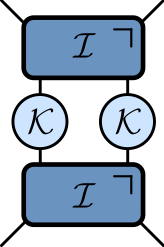\begin{aligned} \mathcal{K} &= \exp\left[-i b X\right]\\ \mathcal{I} &= \exp\left[-iJ Z_1 Z_2 -i \left(h_1 Z_1 + h_2 Z_2\right)/2\right]. \end{aligned}

### Entanglement Growth for Self-Dual KIM

$$\lim_{L\to\infty} S_A =\min(2t-2,N_A)\log 2,$$

• Any $h_j$; initial $Z_j$ product state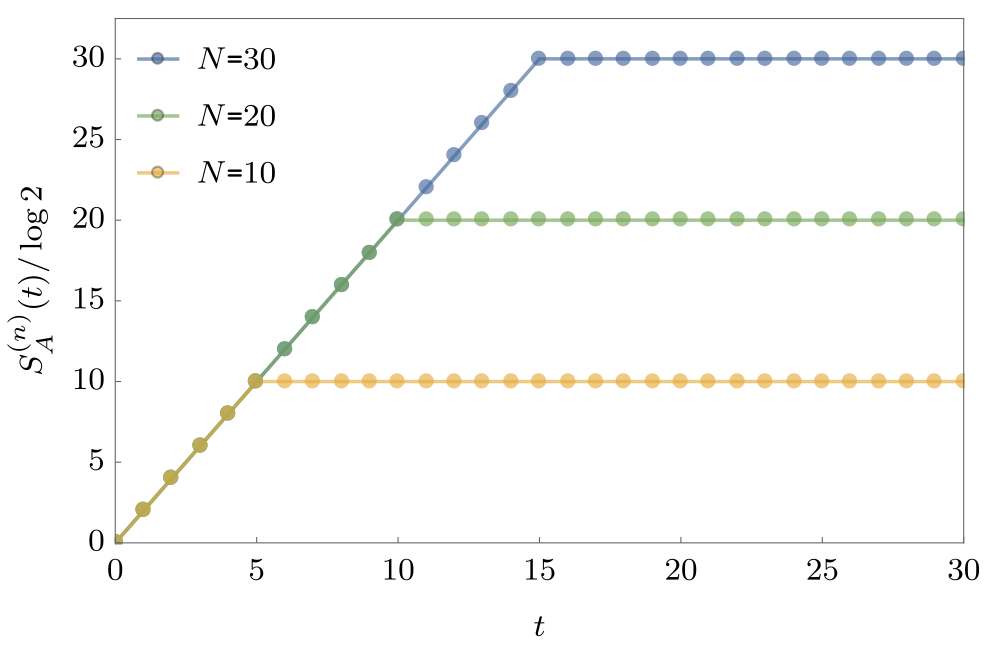### Dual unitarity

• Recall KIM has circuit representation\begin{aligned} \mathcal{K} &= \exp\left[-i b X\right]\\ \mathcal{I} &= \exp\left[-iJ Z_1 Z_2 -i \left(h_1 Z_1 + h_2 Z_2\right)/2\right]. \end{aligned}

• At $|J|=|b|=\pi/4$ model is dual unitary

### ‘KIM’ property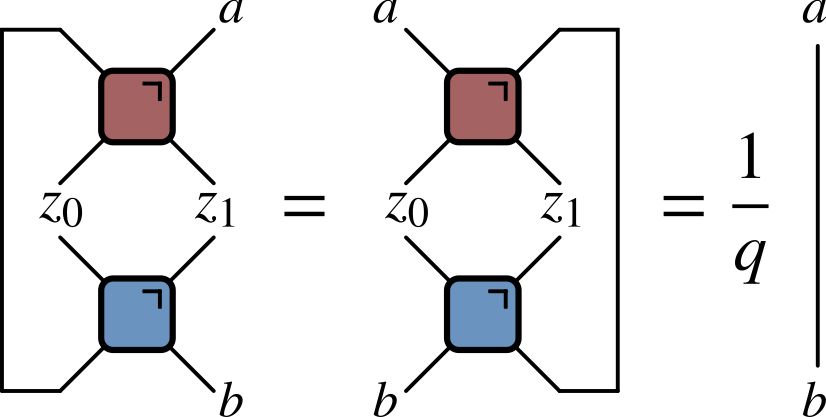• ($q=2$ here) Not satisfied by e.g. $\operatorname{SWAP}$

• Maps product states to maximally entangled (Bell) states

• Product initial states also work for KIM!

• Piroli et al (2020) studied more general initial states

### Correlation functions

• Infinite temperature correlator $\tr\left[\sigma^\alpha_x(x,t)\sigma^\beta(y,0)\right]$

### Krajnik-Prosen model

• Classical circuit

\begin{align*} \Phi_{\tau}\left(\mathbf{S}_{1}, \mathbf{S}_{2}\right) &=\frac{1}{\sigma^{2}+\tau^{2}}\left(\sigma^{2} \mathbf{S}_{1}+\tau^{2} \mathbf{S}_{2}+\tau \mathbf{S}_{1} \times \mathbf{S}_{2}, \sigma^{2} \mathbf{S}_{2}+\tau^{2} \mathbf{S}_{1}+\tau \mathbf{S}_{2} \times \mathbf{S}_{1}\right) \\ \mathbf{S}_1^2&=\mathbf{S}_1^2=1\qquad \sigma^{2} =\frac{1}{2}\left(1+\mathbf{S}_{1} \cdot \mathbf{S}_{2}\right) \end{align*}

### “Space-time duality” of KP model

• $\tilde\Phi_\tau$ coincides with $\Phi_\tau$ after flipping

$$\mathbf{S}_x^t \longrightarrow \tilde{\mathbf{S}}_x^t = (-1)^{x+t+1}\mathbf{S}_x^t$$

### Nonzero correlations in the KP model

Model is not space-time dual in same sense as dual unitary circuits!

## Outline

• Cellular automata: elementary, block, reversible, etc.

• Space-time duality for CA

• Models with continuous state space

• Clifford circuits

### Conway’s Game of Life

1. Any live cell with two or three live neighbours survives
2. Any dead cell with three live neighbours becomes a live cell
3. All other live cells die in the next generation

### Elementary cellular automata

• “Space” is one dimension with cells $x_n=0,1$ $n\in\mathbb{Z}$

• Update cells every time step depending on cells in neighborhood

• Neighborhood is cell and two neighbors for elementary CA

• Update specified by function

$$f:\{0,1\}^3\longrightarrow \{0,1\}.$$

$$x^{t+1}_{n} = f(x^{t}_{n-1},x^{t}_{n},x^{t}_{n+1})$$

• How many possible functions?

### Wolfram’s rules

• Domain of $f$ is $2^3=8$ possible values for three cells

• $2^8=256$ possible choices for the function $f$

• List outputs corresponding to inputs: 111, 110, … 000

111110101100011010001000
01101110
• Interpret as binary number: this one is Rule 110

### Elementary CA

• Many behaviors, from ordered (Rule 18) to chaotic (Rule 30)
• Rule 110 is capable of universal computation!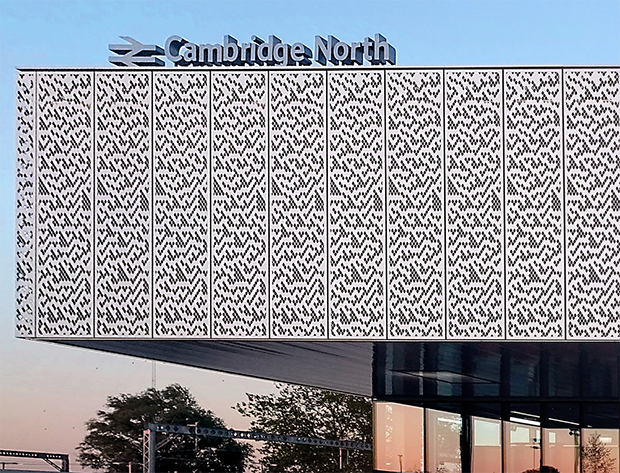### CAs as model physics

• Notion of a causal “light cone” (45 degree lines)

• Variety of possible behaviors: chaos, periodicity, …

### Chaos

• Rapid growth of small differences between two trajectories
• Smallest change: flip one site and monitor $z^t\equiv x^t\oplus y^t$

### Chaos phenomenology

• No exponential growth (c.f. Lyapunov exponent in continuous systems)

• Track number of differences (Hamming distance) between trajectories

• Propagating “front” cannot exceed “speed of light”: generally slower

### Theory?

• No chance of solving the dynamics of any one CA

• Looking for generic properties: natural to consider ensembles

• of initial conditions
• of rules

### Probabilistic CA

• Choose rules iid for each site and instant
• Cell values are now white noise

### Exploring ensemble

• Fluctuations of front are larger and average speed $<$ maximum

• Interesting variation: choose output $1$ with probability $p$

• $p\neq 1/2$ makes dynamics less one-to-one. What happens?

### Phase transition

• For $0.25\lesssim p\lesssim 0.75$ front propagates to infinity

• Outside this region, front dies out

• In finite system two copies always merge after exponentially long time

### Markov chain on $z^t\equiv x^t\oplus y^t$

• $z^{t+1}_{n}=1$ only if at least one of $z^t_{n\pm 1}=1$

### Classical version of MIPT?

• Measurements purify state; analogous to non-injective rules in CA

• It was a surprise that a mixed state survives finite measurement rate

• But… a chaotic front survives non-injective rules (up to a point)

### Reversibility

• No elementary CAs are reversible (bijective)!

• Reversibility is undecidable above one spatial dimension

• $∃$ reversible constructions

### Block cellular automaton

• Partition cells into blocks (Margolus neighborhoods)
• Apply invertible mapping to block
• Alternate overlapping partitions

### Spacetime representation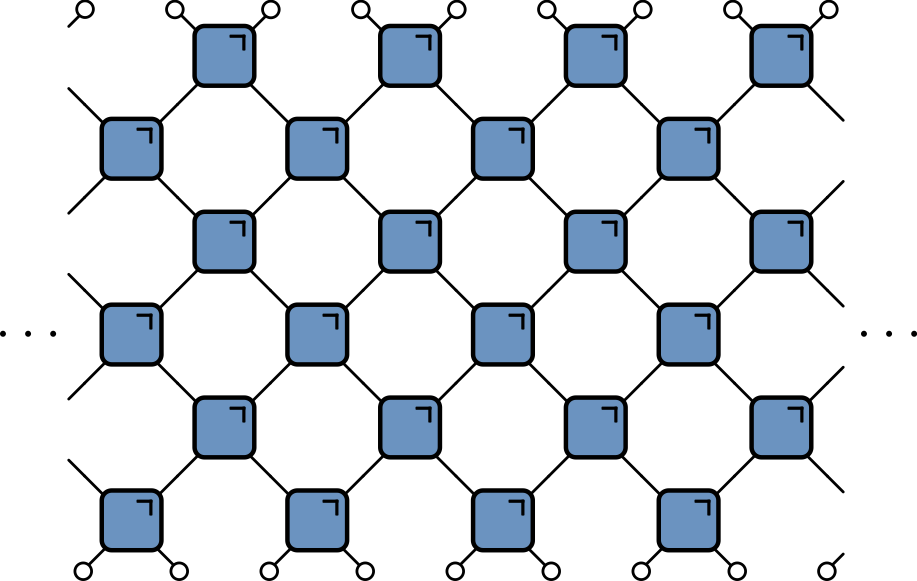• Blue squares: invertible mapping on states of two sites: 00, 01, 10, 11

## 24 reversible models

• Each block a permutation of 00, 01, 10, 11

• $4!=24$ blocks

• Order:

1. (0123)
2. (0132)
3. (0213), and so on
• Block 2 is the map $(00, 01, 10, 11) ⟶ (00, 10, 01, 11)$ (SWAP)

### Ensemble of block CAs

• Results qualitatively similar to chaotic phase of of PCA

• No phase transition because all blocks are reversible

### Circuit notation

$$f:\Sigma\times\Sigma \longrightarrow\Sigma\times\Sigma, \qquad \Sigma=\{0,1\}$$

$$(c,d) = f(a,b)$$

$$F_{ab,cd} = \begin{cases} 1 & \text{if } (c,d) = f(a,b) \\ 0 & \text{otherwise} \end{cases}$$

• If $f(\cdot,\cdot)$ is one-to-one:

$$\sum_{a,b} F_{cd,ab} = \sum_{c,d} F_{cd,ab} = 1$$

• Circle indicates sum over index
• Relaxing 0-1 constraint gives bistochastic matrices

• Every bistochastic matrix is a (nonunique) convex combination of permutations (Birkhoff—von Neumann)

• Interpret Markov chain as probabilistic BCA

### Dual reversibility

• If $(c,d)=f(a,b)$ require bijection $\tilde f$ satisfying $(d,b)=\tilde f(c,a)$
• In terms of $F_{ab,cd}$

$$\sum_{a,c} F_{cd,ab} = \sum_{b,d} F_{cd,ab} = 1$$

### Equivalent formulation

• Write

\begin{align*} f(a,b)=(f_c(a,b),f_d(a,b))\\ \tilde f(c,a)=(\tilde f_d(c,a),\tilde f_b(c,a)) \end{align*}

• Dual reversibility implies that the maps $f_c(a,\cdot)$ and $f_d(\cdot,b)$ are bijections across diagonal

### Three state models

• Of the 24 reversible blocks for two states, 12 are dual reversible

• Three states: Borsi and Pozsgay (2022) find 227 DR models

### The linear block

$$(c,d) = f(a,b) = (a + b, a - b), \mod 3$$

• Original dual unitary circuit from Hosur et al.
• Unusual behavior of recurrence time
• For $L = 2\times 3^m$ have $T_\text{recur}=2L$
• Borsi and Pozsgay prove using Fourier analysis over finite fields

### Origin of “fractal” recurrence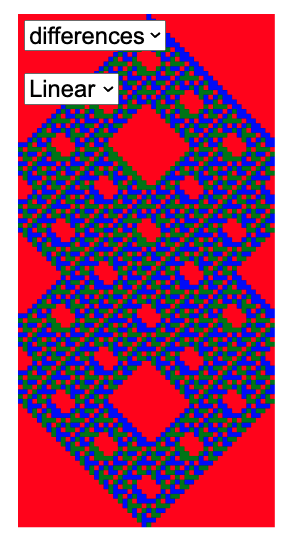$L=54=2\times 3^3$, $T_\text{recur}=2L=108$

### Mutual information

• Disjoint regions $A$ and $\bar A$: how much does one tell about the other?

• Use mutual information: measure of dependence of random variables

• Suggested in this context by Pizzi et al. (2022)

• MI defined as $$I(X;Y) \equiv S(X) + S(Y) - S(X,Y)$$

• $S(X)$ is entropy of $p_X(x)$; marginal distribution of $X$
• $S(Y)$ is entropy of $p_Y(y)$; marginal distribution of $Y$
• $S(X,Y)$ is entropy of joint distribution $p_{(X,Y)}(x,y)$
• Vanishes if $p_{(X,Y)}(x,y)=p_X(x)p_Y(y)$

### Simple example

• Suppose either $X=Y=1$ or $X=Y=0$, with equal probability

\begin{align*} p_{(X,Y)}(0,0)&=p_{(X,Y)}(1,1)=1/2\\ p_{(X,Y)}(1,0)&=p_{(X,Y)}(0,1)=0 \end{align*}

$$I(X;Y)=S(X) + S(Y) - S(X,Y)= 1+1-1=1 \text{ bit}$$

### Toy model (classical reprise)

• Initial distribution factorizes over correlated pairs
• Apply SWAPs
• 1 bit MI for every pair with one member in $A$ and one in $\bar A$

$$I(A;\bar A) = \min(4\lfloor t/2\rfloor, |A|) \text{ bits}$$

• $|A|$ is (even) number of sites in $A$

• Total entropy conserved (c.f Liouville’s theorem)

• Entropy of initial distribution is half max, but entropy $S(A)$ saturates at maximal value (thermalization in time $\sim |A|/2$)

• This model is not so special! Any of the dual reversible BCAs behaves exactly the same!

Graphical proof same as for dual unitaries

• $S(A)$ for 8 central sites
• Marginalize over $\bar A$

• After using dual reversibility, result is reversible automaton applied to initial state with $S(A)=6$ bits

### Models with continuous state space

$$f:\Sigma\times\Sigma \longrightarrow\Sigma\times\Sigma$$

• Reversible: $f$ must be a bijection, so inverse $f^{-1}$ exists

• Probability distribution $p(a,b)$ on two sites is mapped to a distribution $$p_f(c,d) = |\det Df|^{-1} p(f^{-1}(c,d)),$$ $Df$ is Jacobian matrix

• Impose $|Df|=1$ so that uniform (infinite temperature) distribution is preserved

### Landau—Lifshitz circuit

\begin{align*} \Phi_{\tau}\left(\mathbf{S}_{1}, \mathbf{S}_{2}\right) &=\frac{1}{\sigma^{2}+\tau^{2}}\left(\sigma^{2} \mathbf{S}_{1}+\tau^{2} \mathbf{S}_{2}+\tau \mathbf{S}_{1} \times \mathbf{S}_{2}, \sigma^{2} \mathbf{S}_{2}+\tau^{2} \mathbf{S}_{1}+\tau \mathbf{S}_{2} \times \mathbf{S}_{1}\right) \\ \sigma^{2} &:=\frac{1}{2}\left(1+\mathbf{S}_{1} \cdot \mathbf{S}_{2}\right) \end{align*}

• Symplectic map on $S^2\times S^2$

### Dual reversibility

• As before $(d,b) = \tilde f(c,a)$. Require $|D \tilde f|=1$

• Discrete case: bijectivity of $\tilde f$ equivalent to existence of diagonal bijections $f_c(a,\cdot):\Sigma_b\longrightarrow \Sigma_c$ and $f_d(\cdot,b):\Sigma_a\longrightarrow \Sigma_d$

• Continuous case: additional condition: bijections have unit determinant

• Recall $$p_f(c,d) = |\det Df|^{-1} p(f^{-1}(c,d)),$$
• Equivalent to $$p_f(c,d) = \int \delta((c,d)-f(a,b)) p(a,b), d\mu(a) d\mu(b)$$ $$1 = \int \delta((c,d)-f(a,b))\, d\mu(a) d\mu(b)$$
• $|D\tilde f|=1$ guarantees that

$$1 = \int \delta((d,b)-\tilde f(c,a))\, d\mu(a) d\mu(c)$$

• Not analog of
• Even if $(c,d)=f(a,b)$ and $(d,b)=\tilde f(c,a)$: $$\delta((c,d)-f(a,b))\neq \delta((d,b)-\tilde f(c,a))$$

### Necessary condition

$$\delta((c,d)-f(a,b))= \delta((d,b)-\tilde f(c,a))$$

• Requires diagonal bijections satisfy

$$|Df_c(a,\cdot)|=1\qquad |Df_d(\cdot,b)|=1$$

• Not satisfied by Krajnik—Prosen model!

## Symplectic dynamics

• State space $\Sigma$ is symplectic manifold with symplectic form $\omega$

• $f:\Sigma\times\Sigma\longrightarrow\Sigma\times\Sigma$ obeys $f^{*}(\omega_1+\omega_2)=\omega_1+\omega_2$

• $\omega$ has (locally) canonical form

$$\omega = \sum_{i=1}^{n} dx_i\wedge dy_i$$

• $Df$ is symplectic matrix

\begin{align*} Df^T \Omega Df &= \Omega\qquad \Omega \equiv\operatorname{diag}(\omega,\omega)\\ \omega &= \begin{pmatrix} 0 & \mathbb{1}_n \\ -\mathbb{1}_n & 0 \end{pmatrix} \end{align*}

• Rearranging gives condition on spatial Jacobian $D\tilde f$ $$D\tilde f^T\operatorname{diag}(\omega,-\omega) D\tilde f = \operatorname{diag}(-\omega,\omega).$$

• $\tilde f$ not symplectic but may be made so by composing with pair of maps $\tau_{1,2}$ that reverse signs of $\omega_1$ and $\omega_2$ e.g. $\tau_{1,2}$ $y_i\to -y_i$

• $\tau_2\circ \tilde f\circ \tau_1$ is then symplectic

• In Krajnik—Prosen model this corresponds to $$\mathbf{S}_x^t \longrightarrow \tilde{\mathbf{S}}_x^t = (-1)^{x+t+1}\mathbf{S}_x^t$$

• Any symplectic map volume preserving in spatial direction

### Arnold’s Cat map

• Area preserving (symplectic) linear map on torus

$$\mathcal{K}:\begin{pmatrix} x \\ y \end{pmatrix}\longrightarrow \begin{pmatrix} a & 1 \\ ab - 1 & b \end{pmatrix} \begin{pmatrix} x \\ y \end{pmatrix}\qquad \mod 1$$

• Chaotic when one Lyapunov exponent exceeds one for $|a+b|>2$.
• Common choice $a=2$, $b=1$

### Spatiotemporal cat

• Gutkin and Osipov (2016) define map on $N$ copies of torus

• Coupling between sites via \begin{align*} x_{n}&\longrightarrow x_n \\ y_{n}&\longrightarrow y_n - x_{n-1} - x_{n+1} - V'(x_n) \end{align*}\qquad \mod 1

• Generated by Hamiltonian (NB $V(x)$ periodic) $$H_\text{c} = \sum_n \left[x_{n} x_{n+1} + V(x_n)\right]$$

• Alternate with cat maps $\mathcal{K}_n$ on each site

### Circuit form

• On neighbouring sites define

\mathcal{I}_{n,n+1}:\qquad\begin{align} x_{n}&\longrightarrow x_n,\qquad x_{n+1}\longrightarrow x_{n+1} \\ y_{n}&\longrightarrow y_n - x_{n+1}-V'(x_n)/2\qquad y_{n+1}\longrightarrow y_{n+1} - x_{n}-V'(x_{n+1})/2,\qquad \mod 1, \end{align}• Satisfies dual reversible property!

### Lagrangian pictue

• “Momenta” $y_n$ can be eliminated to give two-step (Lagrangian) recurrence for $x_{n,t}$: $$[\Delta x]_{n,t} = (a+b-4)x_{n,t} - V'(x_{nt})-m_{n,t} \mod 1$$ winding numbers $m_{n,t}$ chosen to ensure $x_{n,t}$ stays in the unit interval and $\Delta$ is the 2D Laplacian

• Symmetry between space and time is evident (but not necessary for dual reversibility)

### Vary coupling ($V(x)=0$)

$$H_\text{c} = J\sum_n x_{n} x_{n+1} \qquad J\in \mathbb{Z}$$

$$\begin{pmatrix} x_n \\ y_n \\ x_{n+1} \\ y_{n+1} \end{pmatrix}\longrightarrow \begin{pmatrix} a & 1 & -J & 0 \\ J^2 + ab - 1 & b & -J(a+b) & -J \\ -J & 0 & a & 1 \\ -J(a+b) & -J & J^2 + ab -1 & b \end{pmatrix}\begin{pmatrix} x_n \\ y_n \\ x_{n+1} \\ y_{n+1} \end{pmatrix}$$

• Off-diagonal blocks have det $J^2$; diagonals $1-J^2$

### Clifford circuits and symplectic CA (Schlingemann et al (2008))

• Local space $\mathbb{C}^p$ ($p$ prime): $X$ (“shift”) and $Z$ (“clock”) $$X\ket{q} = \ket{q+1},\qquad Z\ket{q}=e^{2\pi iq/p}\ket{q}$$

• $w(r,k) \equiv X^rZ^k$ satisfy

$$w(r_1,k_1)w(r_2,k_2) = e^{2\pi i(r_1k_2-r_2k_1)/p}w(r_2,k_2)w(r_1,k_1).$$

• Clifford unitary maps $w(r,k)\to w(r',k')$ $$U_\text{c} w(r,k)U^\dagger_{c} = e^{i\theta(r,k)}w(r',k')$$

$$r_1k_2-r_2k_1 = r'_1k'_2-r'_2k'_1, \mod p$$

• If $$r_1k_2-r_2k_1 = r'_1k'_2-r'_2k'_1, \mod p$$ $$\begin{pmatrix} r' \\ k' \end{pmatrix} =\begin{pmatrix} a & b \\ c & d \end{pmatrix}\begin{pmatrix} r \\ k \end{pmatrix},\mod p$$ with $ad-bc=1 \mod p$. Matrix $\in\text{Sp}(2,\mathbb{Z}_p)$.

• For $N$ sites have $w_N(\textbf{r}, \textbf{k})\equiv\prod_{n=1}^N X^{r_n}Z^{k_n}$, and Clifford unitaries on $N$ sites give symplectic matrix $\text{Sp}(2N,\mathbb{Z}_p)$

• Circuit made of Clifford unitary blocks can therefore be specified by gates taken from $\text{Sp}(4,\mathbb{Z}_p)$.

### Sommers et al automata

• Correlations $\tr\left[\sigma^a_x(x,t)\sigma^b(y,0)\right]$ vanish

• OTOC has maximal speed and doesn’t broaden (Claeys and Lamacraft (2020))